# Test: Line Charts- 1

## 5 Questions MCQ Test Logical Reasoning (LR) and Data Interpretation (DI) | Test: Line Charts- 1

Description
Attempt Test: Line Charts- 1 | 5 questions in 10 minutes | Mock test for Banking Exams preparation | Free important questions MCQ to study Logical Reasoning (LR) and Data Interpretation (DI) for Banking Exams Exam | Download free PDF with solutions
QUESTION: 1

### Study the following line graph and answer the questins. Exports from Three Companies Over the Years (in Rs. crore)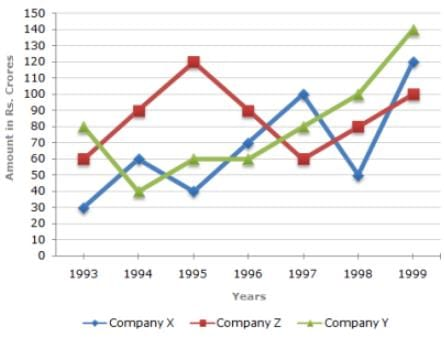Q. For which of the following pairs of years the total exports from the three Companies together are equal?

Solution:

Total exports of the three Companies X, Y and Z together, during various years are:

In 1993 = Rs. (30 + 80 + 60) crores = Rs. 170 crores.

In 1994 = Rs. (60 + 40 + 90) crores = Rs. 190 crores.

In 1995 = Rs. (40 + 60 + 120) crores = Rs. 220 crores.

In 1996 = Rs. (70 + 60 + 90) crores = Rs. 220 crores.

In 1997 = Rs. (100 + 80 + 60) crores = Rs. 240 crores.

In 1998 = Rs. (50 + 100 + 80) crores = Rs. 230 crores.

In 1999 = Rs. (120 + 140 + 100) crores = Rs. 360 crores.

Clearly, the total exports of the three Companies X, Y and Z together are same during the years 1995 and 1996.

QUESTION: 2

### Study the following line graph and answer the questins. Exports from Three Companies Over the Years (in Rs. crore)Q. Average annual exports during the given period for Company Y is approximately what percent of the average annual exports for Company Z?

Solution:

Analysis of the graph: From the graph it is clear that

1. The amount of exports of Company X (in crore Rs.) in the years 1993, 1994, 1995, 1996, 1997, 1998 and 1999 are 30, 60, 40, 70, 100, 50 and 120 respectively.

2. The amount of exports of Company Y (in crore Rs.) in the years 1993, 1994, 1995, 1996, 1997, 1998 and 1999 are 80, 40, 60, 60, 80, 100 and 140 respectively.

3. The amount of exports of Company Z (in crore Rs.) in the years 1993, 1994, 1995, 1996, 1997, 1998 and 1999 are 60, 90,, 120, 90, 60, 80 and 100 respectively.

Average annual exports (in Rs. crore) of Company Y during the given period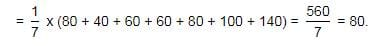Average annual exports (in Rs. crore) of Company Z during the given period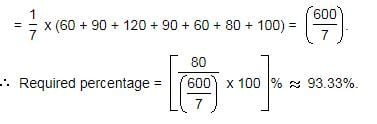QUESTION: 3

### Study the following line graph and answer the questins. Exports from Three Companies Over the Years (in Rs. crore)Q. In which year was the difference between the exports from Companies X and Y the minimum?

Solution:

The difference between the exports from the Companies X and Y during the various years are:

In 1993 = Rs. (80 - 30) crores = Rs. 50 crores.

In 1994 = Rs. (60 - 40) crores = Rs. 20 crores.

In 1995 = Rs. (60 - 40) crores = Rs. 20 crores.

In 1996 = Rs. (70 - 60) crores = Rs. 10 crores.

In 1997 = Rs. (100 - 80) crores = Rs. 20 crores.

In 1998 = Rs. (100 - 50) crores = Rs. 50 crores.

In 1999 = Rs. (140 - 120) crores = Rs. 20 crores.

Clearly, the difference is minimum in the year 1996.

QUESTION: 4

Study the following line graph and answer the questins.
Exports from Three Companies Over the Years (in Rs. crore)Q. What was the difference between the average exports of the three Companies in 1993 and the average exports in 1998?

Solution:

Average exports of the three Companies X, Y and Z in 1993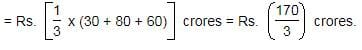Average exports of the three Companies X, Y and Z in 1998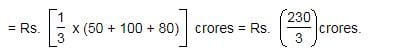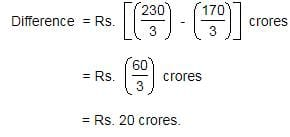QUESTION: 5

Study the following line graph and answer the questins.
Exports from Three Companies Over the Years (in Rs. crore)Q. In how many of the given years, were the exports from Company Z more than the average annual exports over the given years?

Solution:

Average annual exports of Company Z during the given period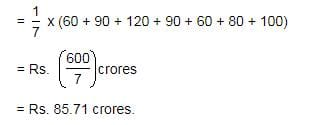From the analysis of graph the exports of Company Z are more than the average annual exports of Company Z (i.e., Rs. 85.71 crores) during the years 1994, 1995, 1996 and 1999, i.e., during 4 of the given years.Use Code STAYHOME200 and get INR 200 additional OFF Use Coupon Code